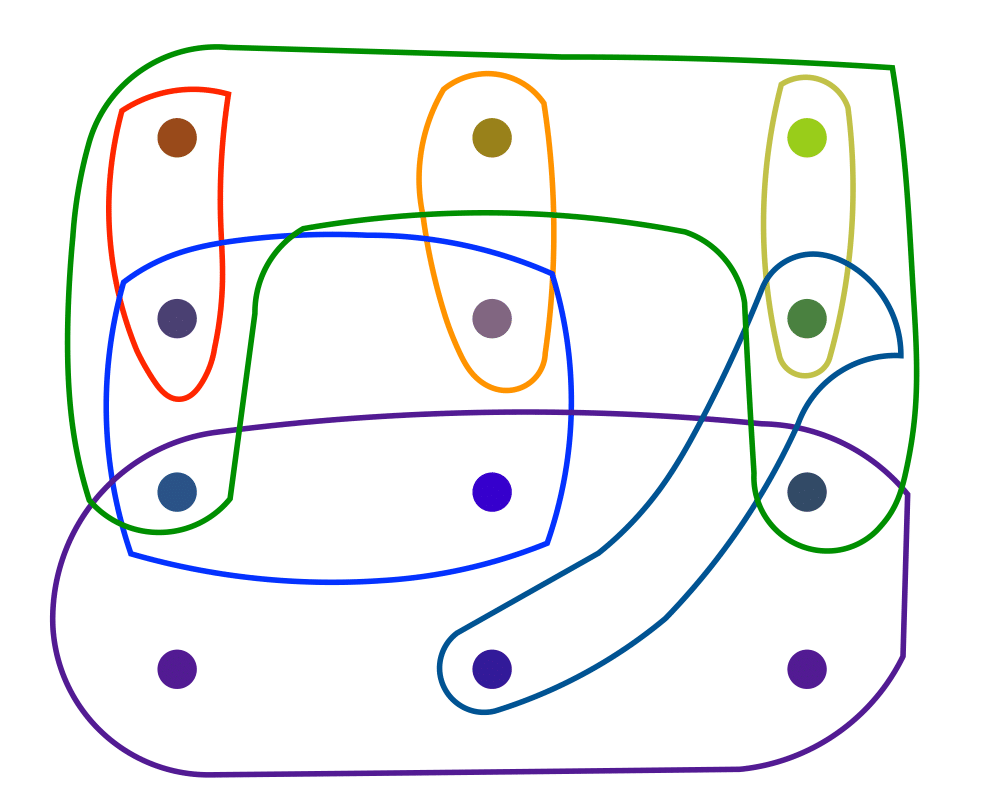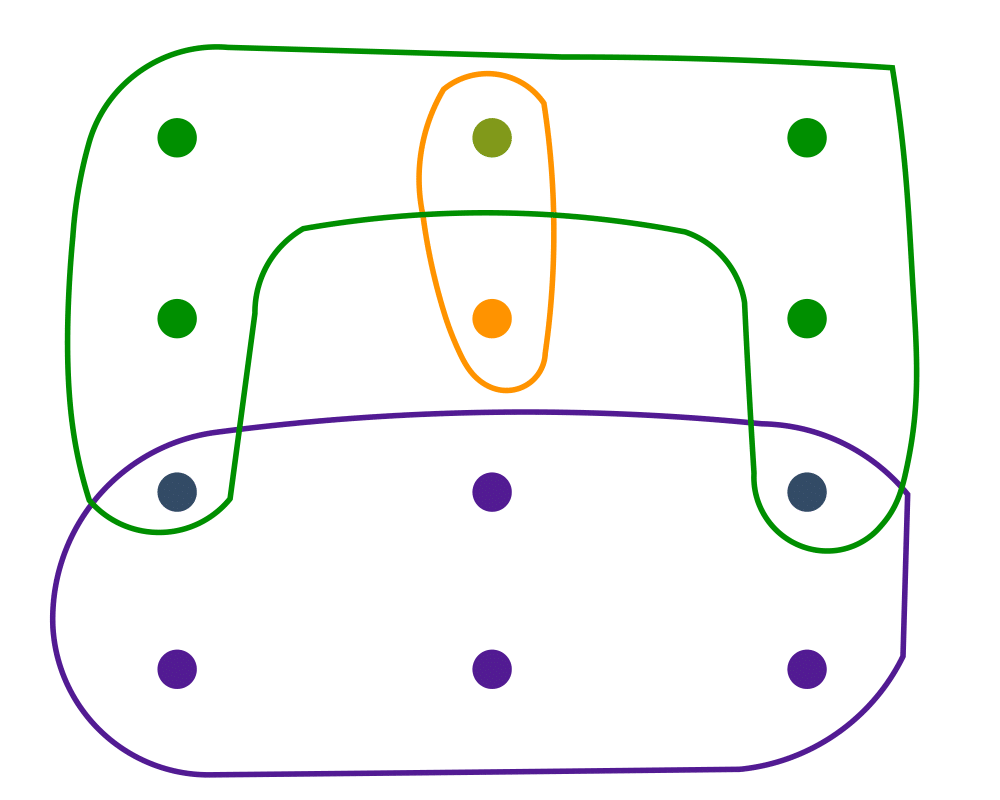Algorithm Repository

# Set CoverInputOutput

Input Description: A set of subsets $$S_1, ..., S_m$$ of the universal set $$U = \{1,...,n\}$$.
Problem: What is the smallest subset of subsets $$T \subset S$$ such that $$\cup_{t_i \in T} t_i = U$$?

Excerpt from The Algorithm Design Manual: Set cover arises when you try to efficiently acquire or represent items that have been packaged in a fixed set of lots. You want to obtain all the items, while buying as few lots as possible. Finding a cover is easy, because you can always buy one of each lot. However, by finding a small set cover you can do the same job for less money.

An interesting application of set cover is Boolean logic minimization. We are given a particular Boolean function of $$k$$ variables, which for each of the $$2k$$ possible input vectors describes whether the desired output is $$0$$ or $$1$$. We seek the simplest circuit that exactly implements this function. One approach is to find a disjunctive normal form (DNF) formula on the variables and their complements, such as $$x1 \bar x2 + \bar{x}1 \bar{x}2$$. We could build one and'' term for each input vector and then or'' them all together, but we might save considerably by factoring out common subsets of variables. Given a set of feasible and'' terms, each of which covers a subset of the vectors we need, we seek to `or'' together the smallest number of terms that realize the function. This is exactly the set cover problem.

Go To Main Page